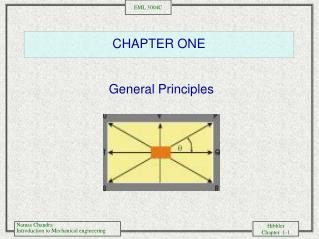DownloadDownload PresentationCHAPTER ONE

# CHAPTER ONE

Télécharger la présentation## CHAPTER ONE

- - - - - - - - - - - - - - - - - - - - - - - - - - - E N D - - - - - - - - - - - - - - - - - - - - - - - - - - -
##### Presentation Transcript

1. CHAPTER ONE General Principles

2. Statics, Dynamics and Mechanics of Materials • Statics deals with the state of rest or motion of bodies with constant velocities • In dynamics, the bodies are allowed to accelerate. • Two Branches of static equilibrium • Statics • Mechanics of Materials • Statics • Equilibrium of Bodies-material independent, uses only static equations • Mechanics of Materials • Relationship between the external loads, the intensity of internal forces and its deformation response

3. Quantities Length (location, position, size) Time (succession of events) Force (Push, Pull) Mass (Properties of Matter) ONLY THREE OF FOUR NEEDED (NEWTONS LAW) Idealization Particle (neglect, size, geometry) Rigid Body (all points within remain in the same position, at fixed distances from each other) Concentrated Force ( over a very small area, zero) Basic Concepts

4. Physical Quantities • Physical Qualities • Mass, • Force • time • distance, • Density • Temperature • Volume • area • length • displacement • velocity • acceleration

5. R R Scalar and Vector Quantities • Scalar Quantities • Described by their magnitude, mass • (italic form) or lower case (a for A) • Vector Quantities • Described by a magnitude, a direction, and a point of application • (Bold Face) in the book • Bar or Arrow in handwritten work • Magnitude or A (italic) or a =

6. Newton’s Laws • Newton’s First Law • A body at rest tends to remain at rest & a body in motion at a constant velocity will tend to maintain the velocity. • Newton’s Second Law • Change of motion is proportional to the moving force impressed and takes place in the direction of the straight line in which such force is impressed.

7. Newton’s Laws • Consequences of Newton’s Third Law • When two bodies interact, a pair of equal and opposite reaction forces will exist at their contact point. • This force pair will have the same magnitude and acts along the same direction, but have opposite sense. • The mutual force of action and reaction between two bodies are equal, opposite, and collinear. Kg = mass=[m] Newton = Force=[F] Slug= mass =[m] lbf = Force = [F]

8. Gravitational Law • Gravitational Law G = universal constant of gravitation m1,m2 = mass of each of the two particles r= distance

9. What is weight? • Weight If = mass of the particle = mass of the earth r = distance of the particle to the earth’s center W = weight of the particle if

10. SI (International System of Units) Meter (m) Second (sec) Kilogram (kg) Newton (N) Units Length, time, mass, force -basic quantities Units (Note: we use bars to denote forces or vectors) Ex : mass = 1kg W = 9.81N

11. Conversion of Units • Conversion of Units FPSSI Force 1 lb = 4.4482 N Mass 1 slug = 14.5938 kg Length 1 ft = 0.3048 m Ex:

12. CHAPTER ONE.. concludes General Principles